# RS Aggarwal Solutions for Class 8 Maths Chapter 2 - Exponents Exercise 2A

Students can refer and download the RS Aggarwal Solutions for the Exercise 2A of Class 8 Maths Chapter 2, Exponents from the link available here. RS Aggarwal Solutions for Class 8 Maths Chapter 2 has 3 exercises. The questions present in the Exercise 2A have been solved by BYJU’S experts in Maths, and this will help you in solving the problems without any obstacles. This exercise helps the students to understand the concepts of Exponents as well as the laws of exponents.

By practising the RS Aggarwal Solutions for Class 8, students will be able to grasp the concepts perfectly. It also helps in boosting their confidence, which plays an important role in their examinations. Hence, students whose aim is to score high in the examinations are advised to go through RS Aggarwal Solutions for Class 8.

## Download PDF of RS Aggarwal Solutions for Class 8 Maths Chapter 2 – Exponents Exercise 2A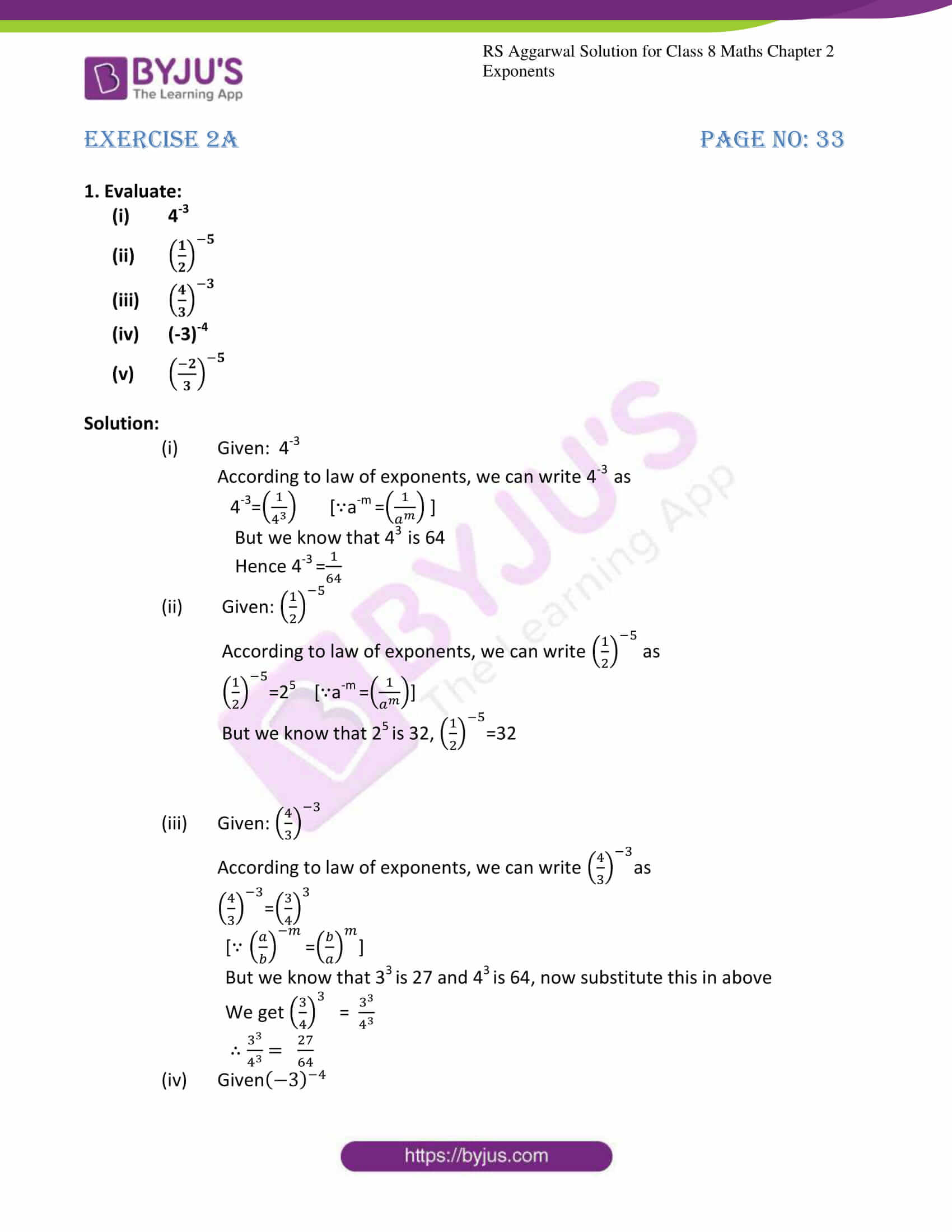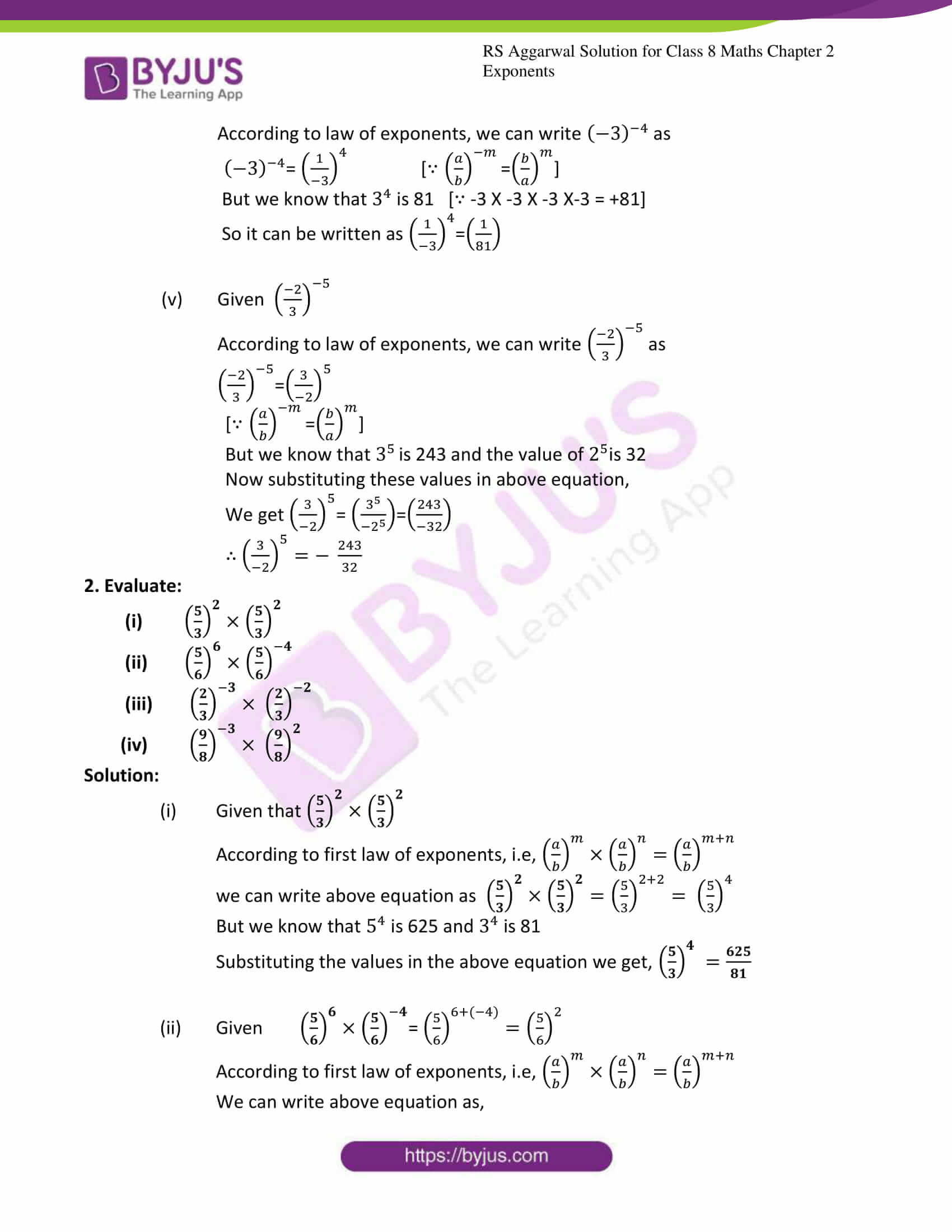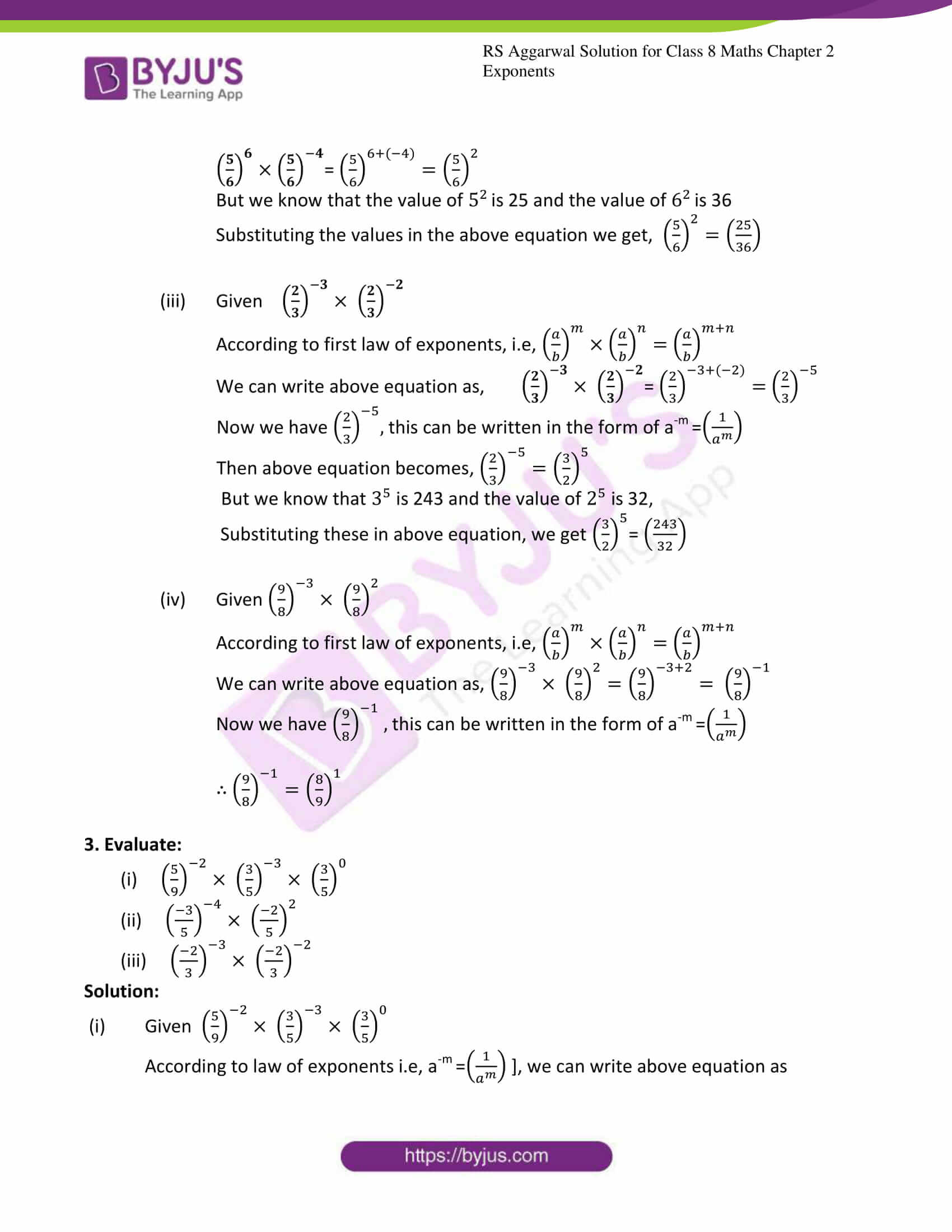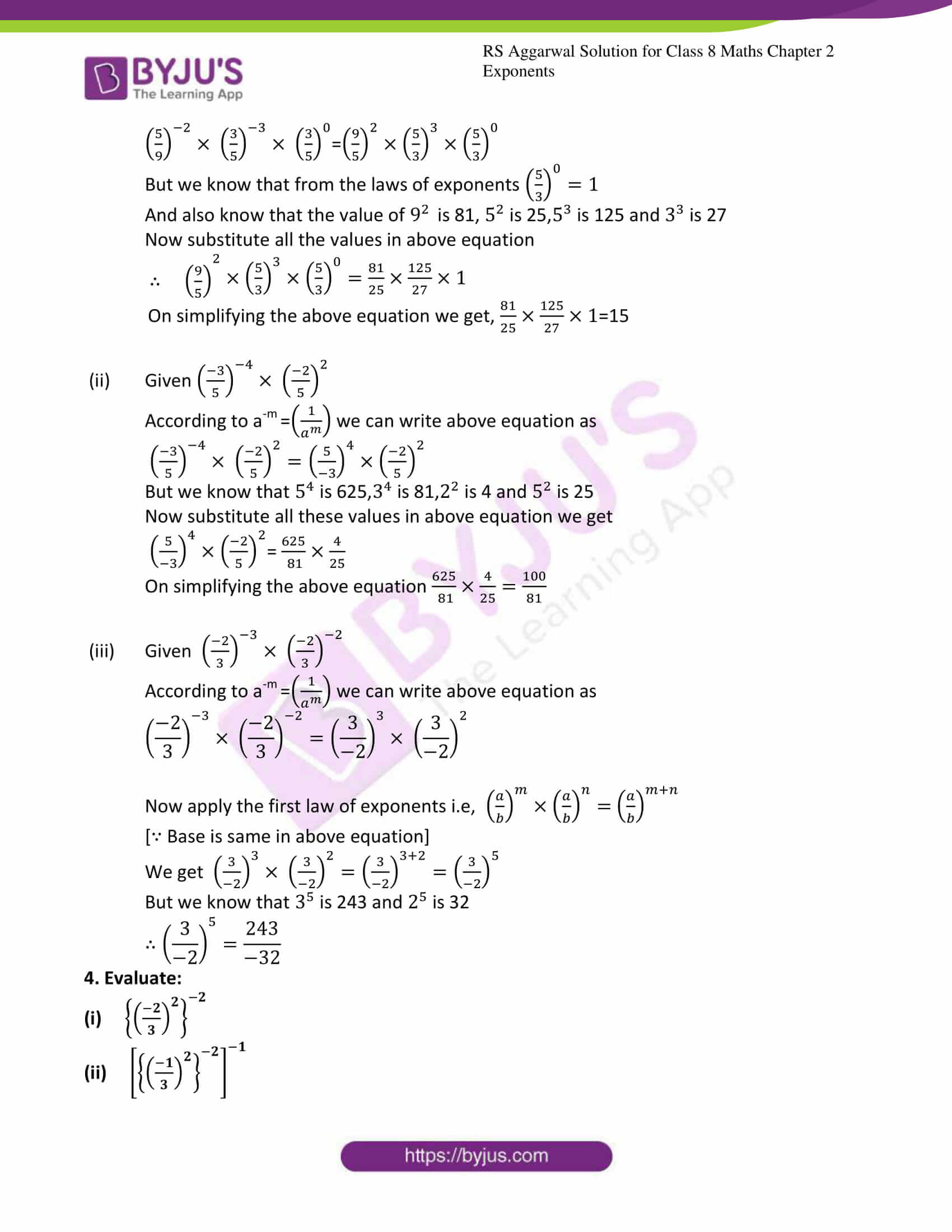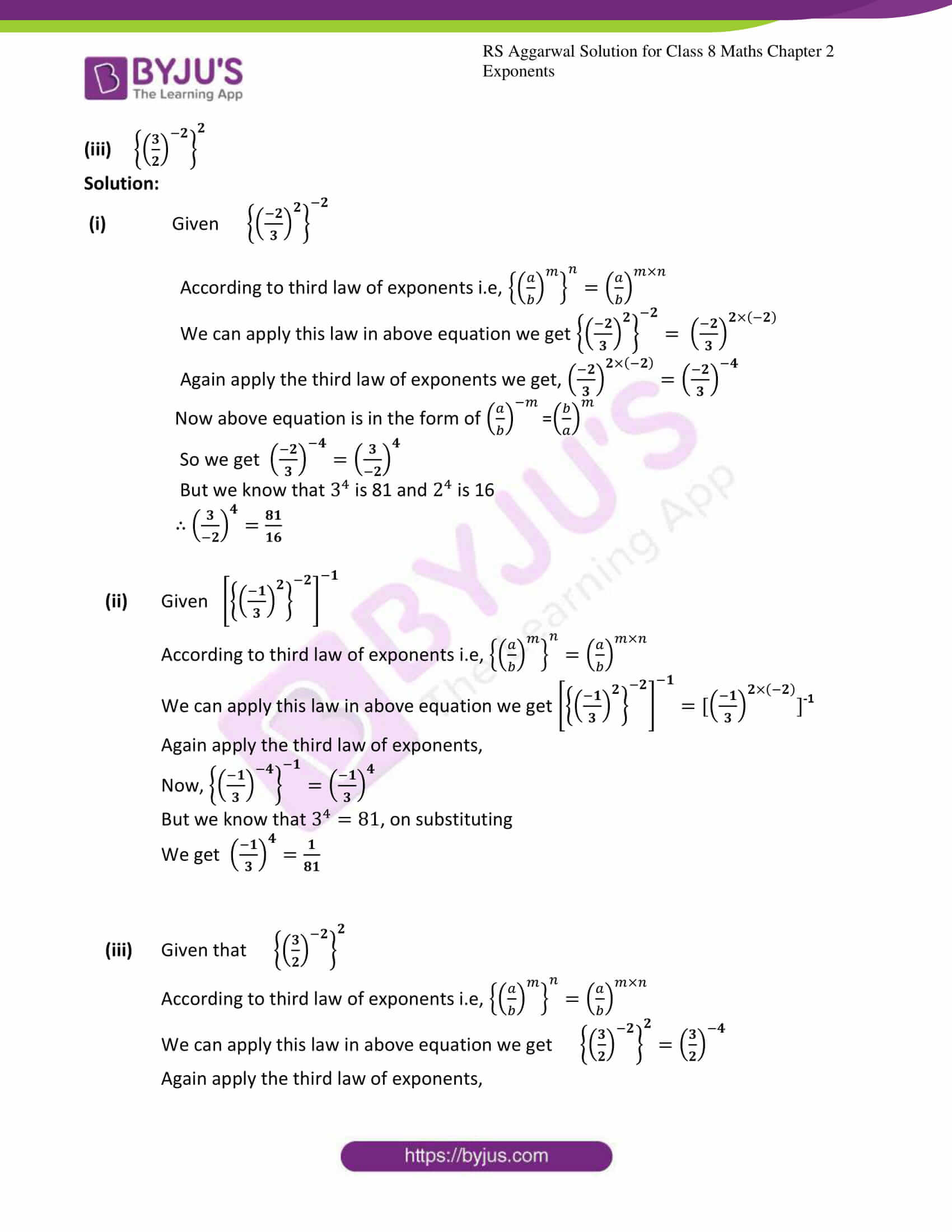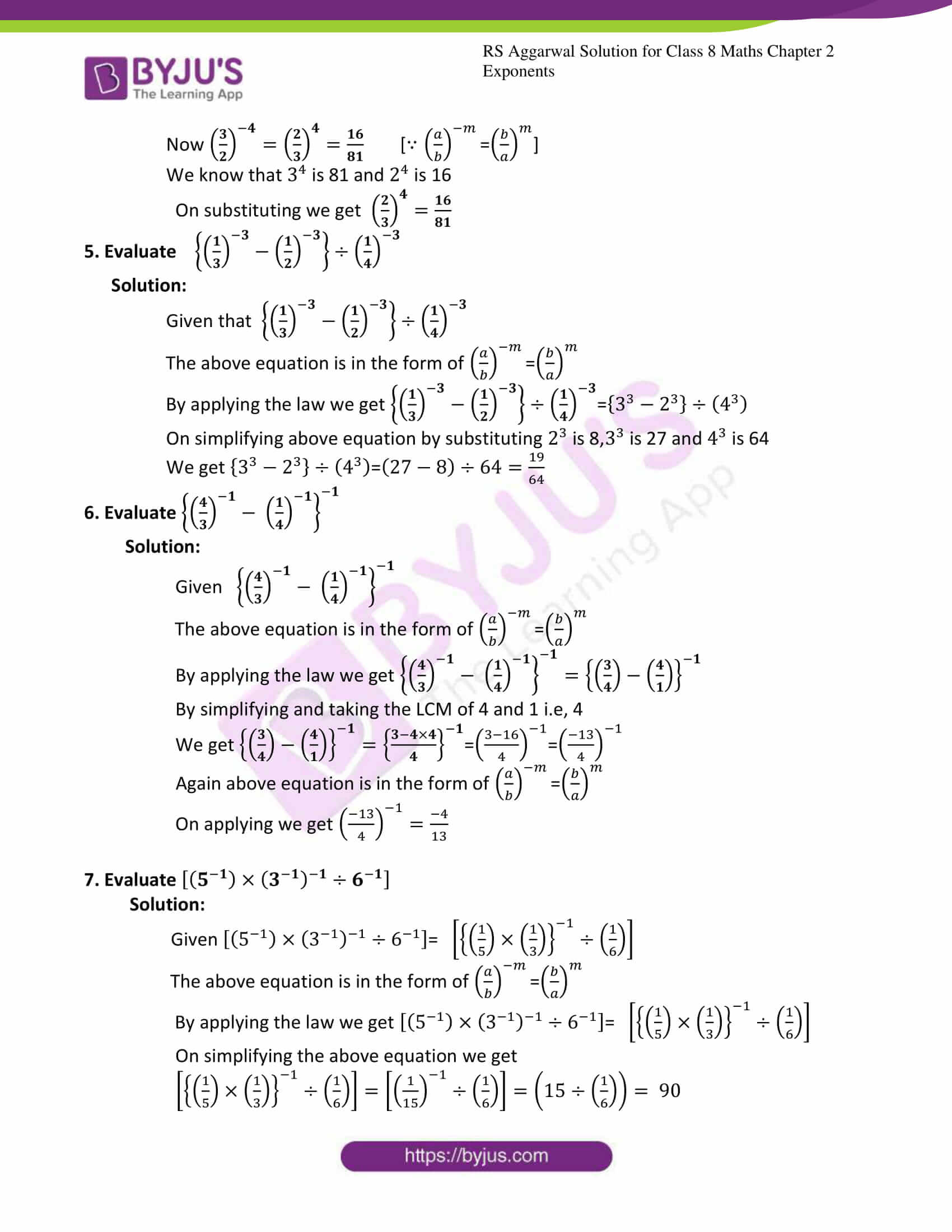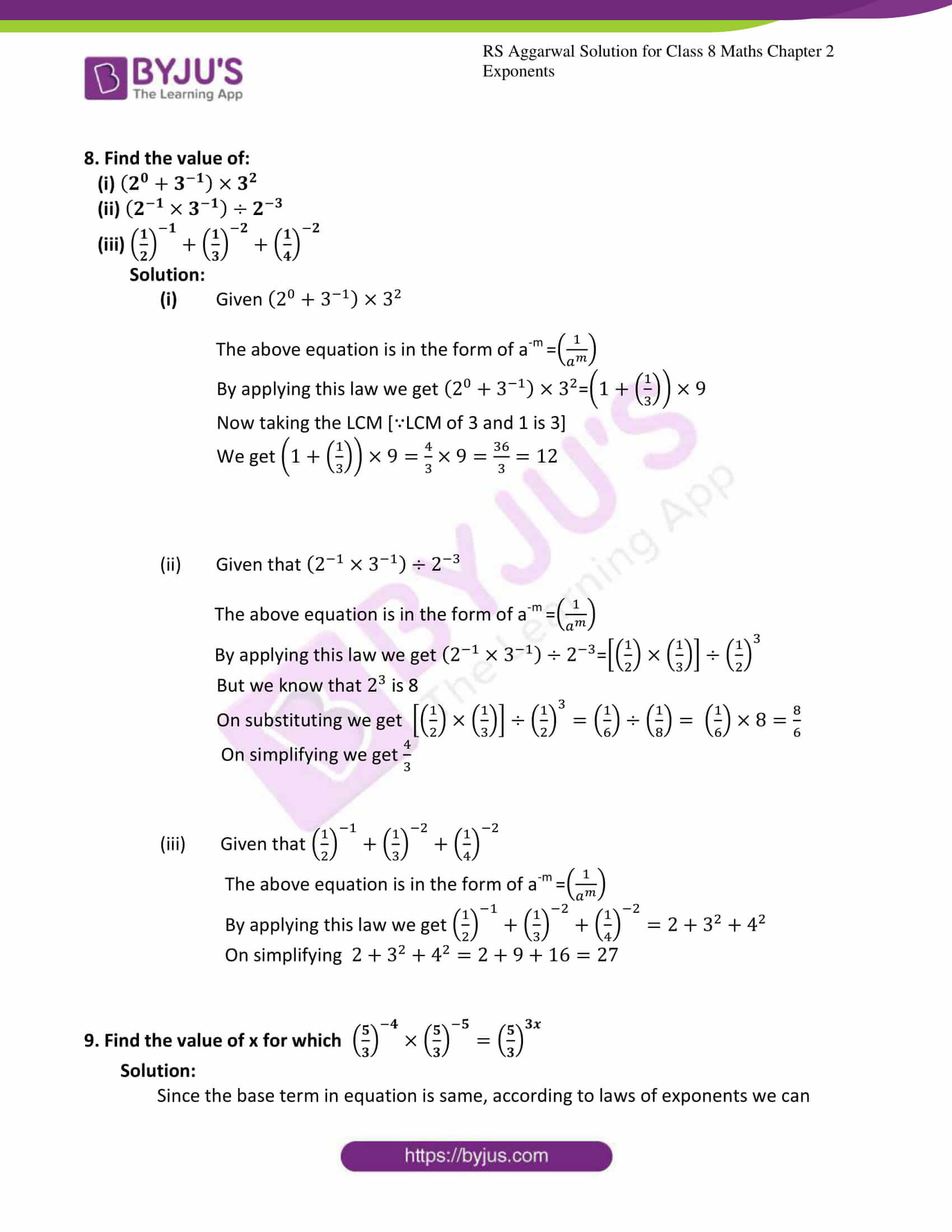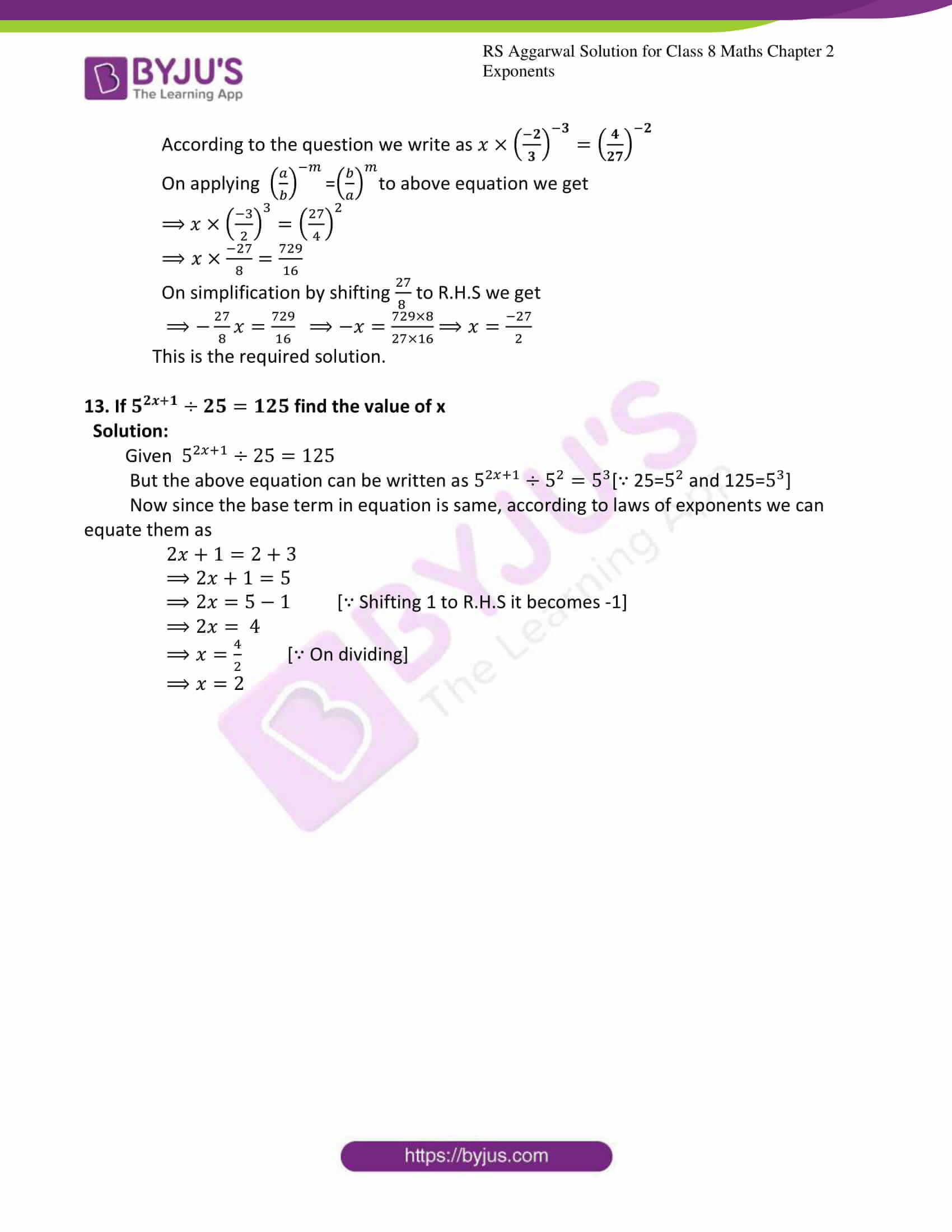### Exercise 2A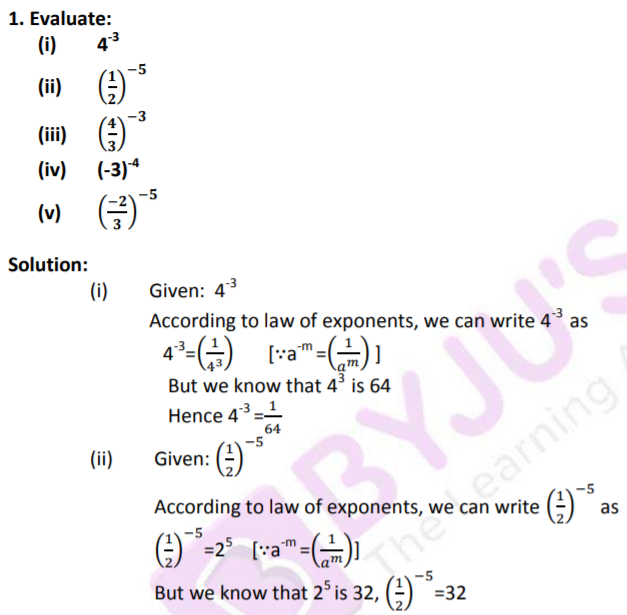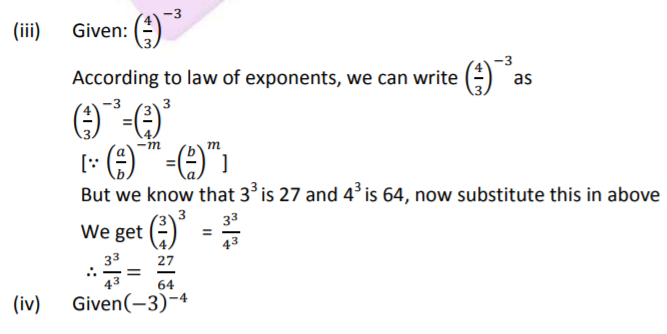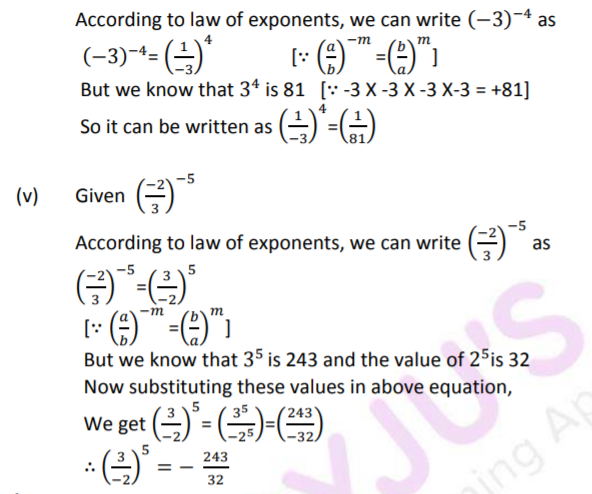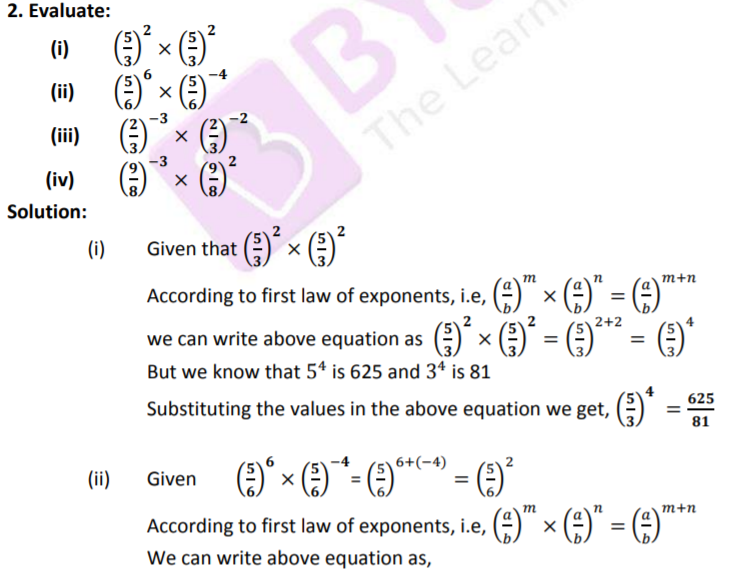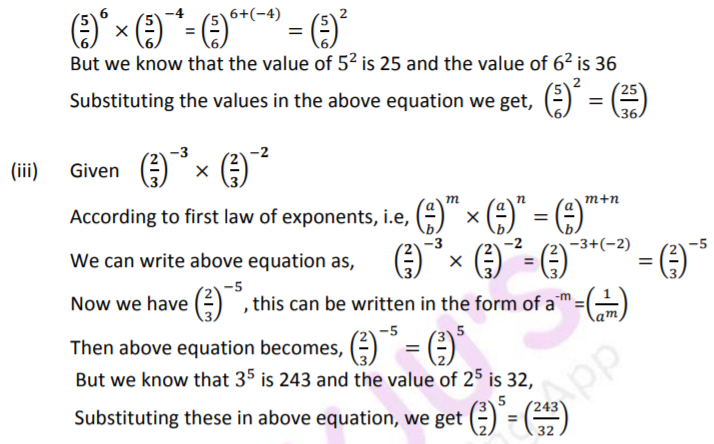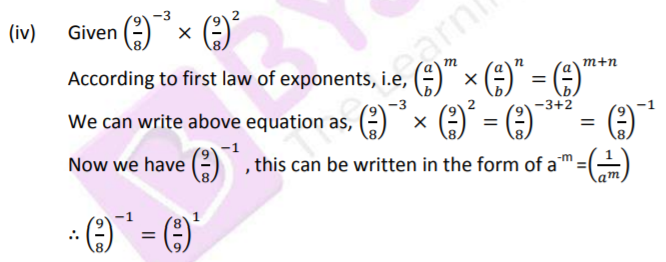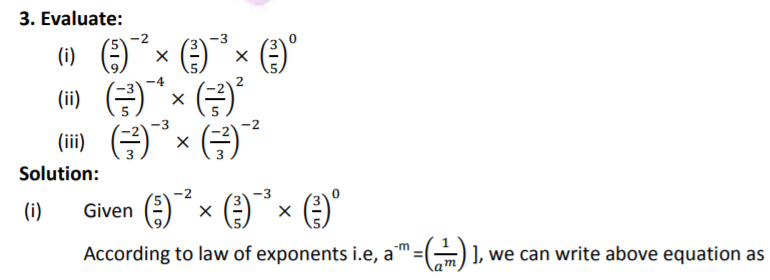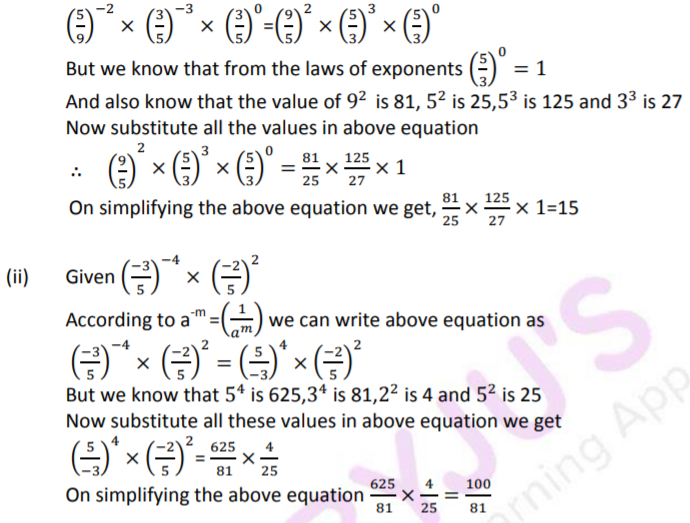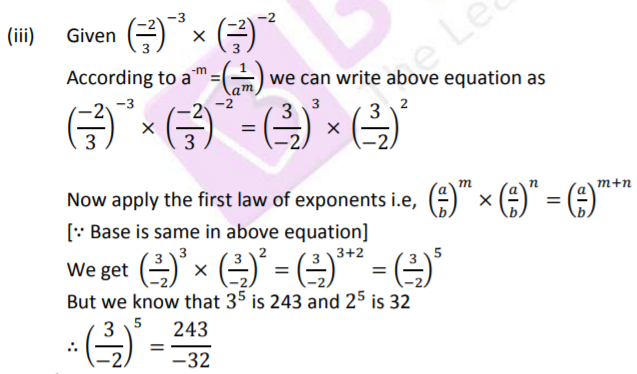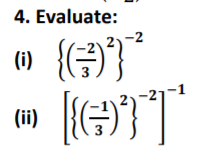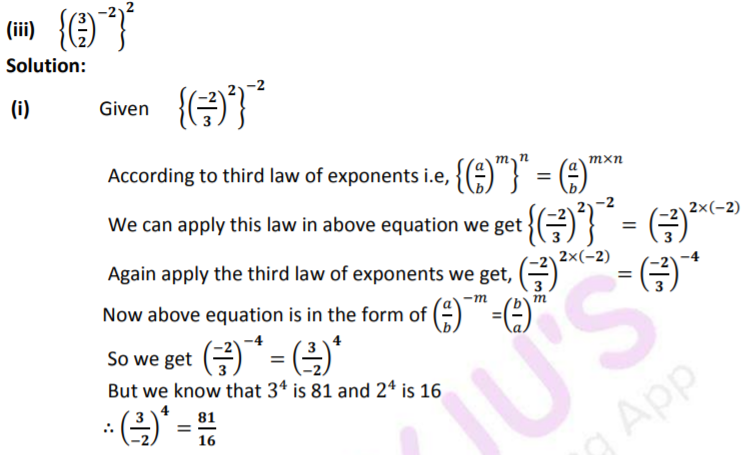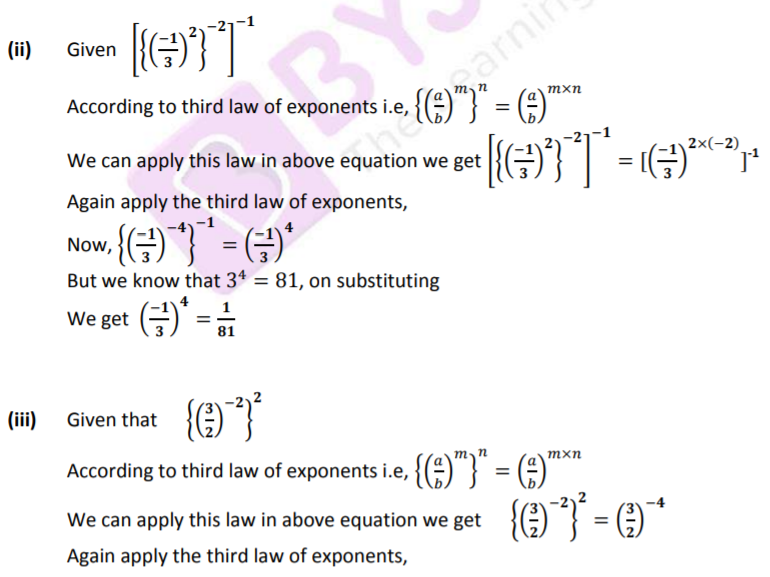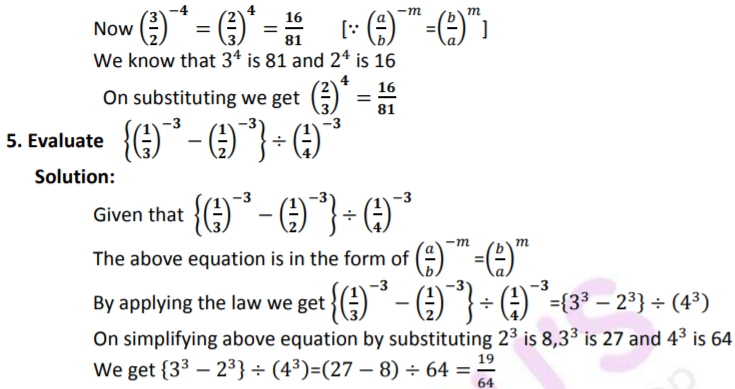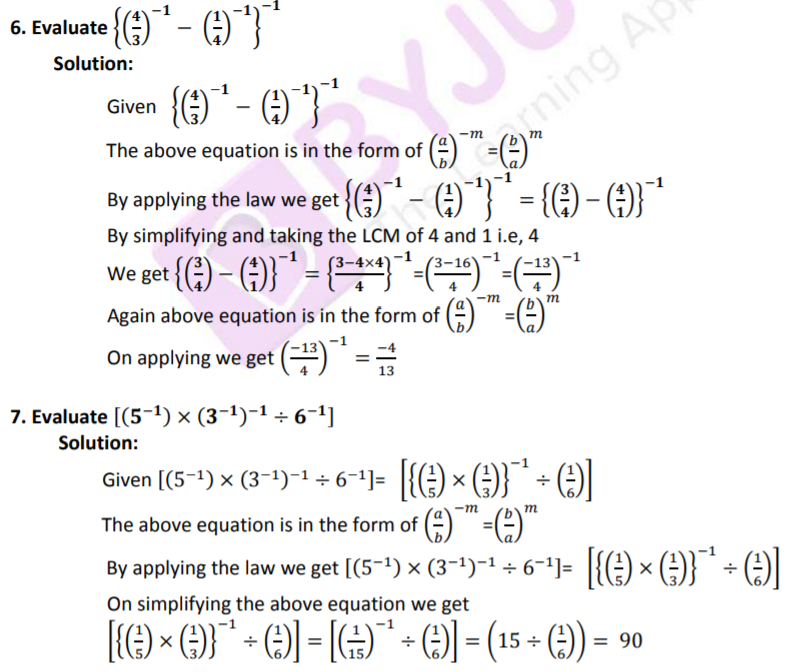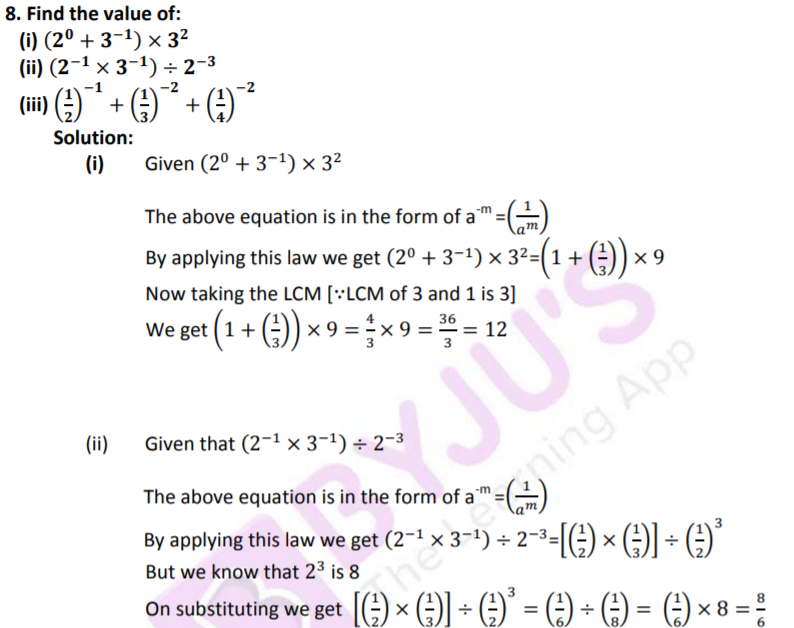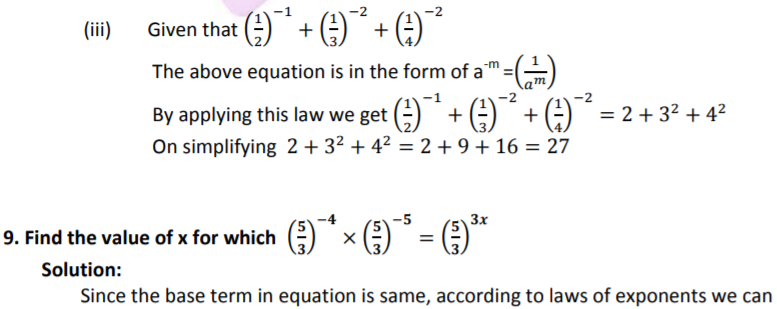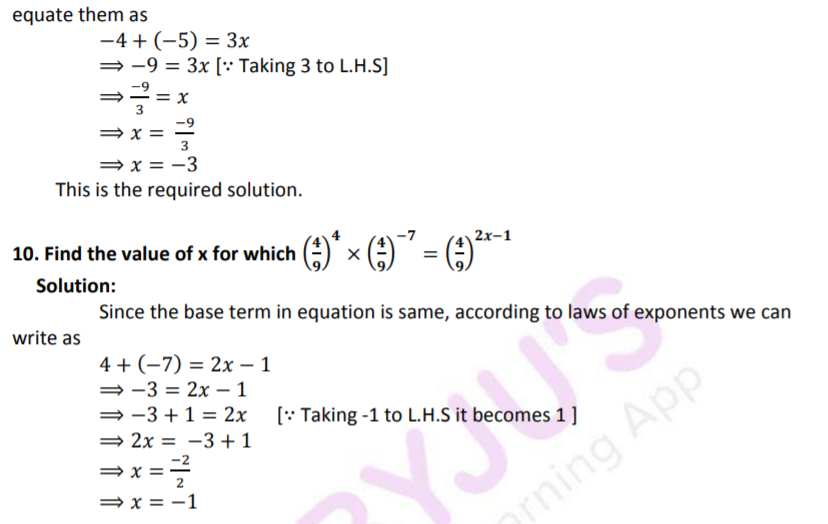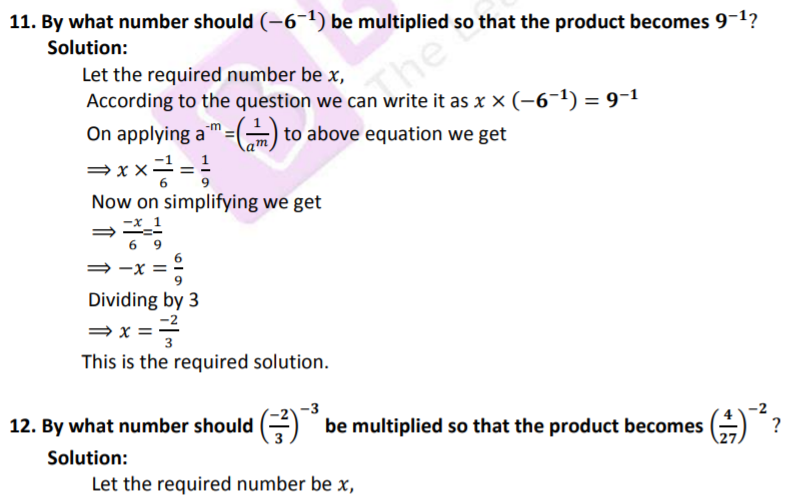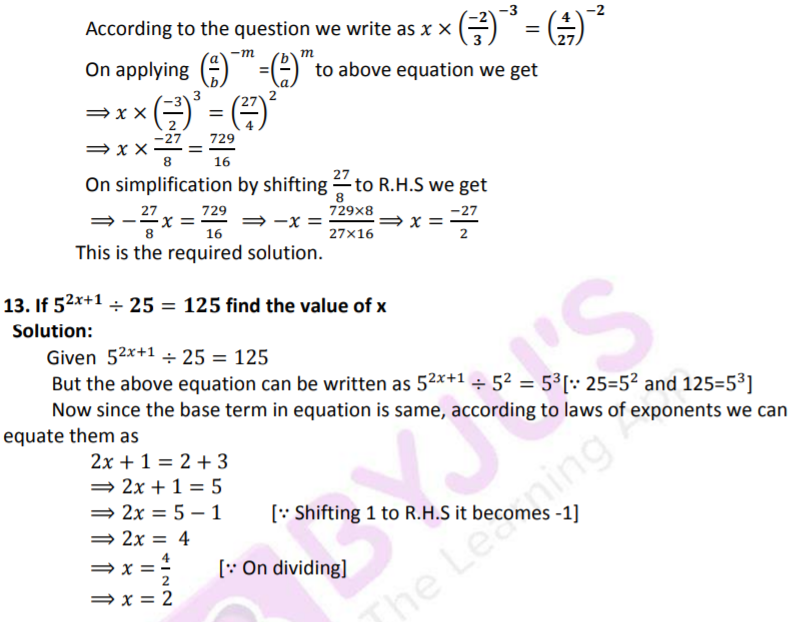### Access other exercises of RS Aggarwal Solutions for Class 8 Maths Chapter 2 – Exponents

Exercise 2B Solutions 7 questions

Exercise 2C Solutions 17 questions

## RS Aggarwal Solutions for Class 8 Maths Chapter 2 – Exponents Exercise 2A

Exercise 2A of RS Aggarwal Solutions for Chapter 2, Exponents, deals with the basic concepts related to Exponents. We can say that this exercise mainly deals with the laws of exponents that the students have learned in this Chapter. Some of the topics focused prior to this exercise include the following.

• Positive integral exponent of a rational number
• Negative integral exponent of a rational number
• Laws of exponents

The RS Aggarwal Solutions can help the students in practising and learning each and every concept as it provides solutions to all questions asked in the RS Aggarwal textbook. Those who aim to score high in the Maths of Class 8 are advised to practice all the questions present in RS Aggarwal as many times as possible.